Thermos Index

Thermodynamics Fundamentals

Work..... Energy..... Heat..... System..... Boundary..... Surroundings..... State..... Property.....
Process..... Reversible / Irreversible processes..... Ideal Gas..... Perfect Gas..... Semi Perfect Gas..... Thermal Efficiency.....

Introduction

Work

Work is defined simply as the product of force (SI units = newtons) and the distance moved in the direction of the force (SI units = metres).  If a block is moved against a constant frictional force of 1 N through a distance of 1 m then 1 N.m (= 1 Joule), of work has been exerted in moving the block.....

Energy

Energy at is simplest level is the capacity of a system to do work.   A compressed spring has potential energy because, when released it can exert, a force over a distance until the spring has is no longer compressed.

A water in motion possesses kinetic energy which can be used to do work using a water turbine..

Potential energy is the form of energy which is stored e.g a body has potential energy due to its position in a gravitation field.  Kinetic energy is the form of energy a body possesses because of its motion.

Energy can have many different forms including

 Thermal energy: sub-microscopic particles in motion. (form of kinetic energy) Mechanical energy: macroscopic objects in motion. (form of kinetic energy) Electrical energy: movement of electrons through a conductor.(form of kinetic energy) Sound energy: compression/expansion of spaces between molecules. (form of kinetic energy) Chemical potential energy: position of electrons relative to atomic nuclei in bound atoms. (form of potential energy) Gravitational energy: position of an object in a gravitational field. (form of potential energy) Electrostatic energy: relative position of charged particles.(form of potential energy)

The total stored energy of a body, substance or system is a property and is given the symbol E and is generally used not as an absolute value but as measure of change between different states ΔE.  The change of internal energy can be obtained from measurements of heat and work.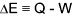The stored energy of a body, substance or system which is independent of electricity, atomic, sound, magnetism, surface tension, motion and gravity is called the internal energy is given the symbol U.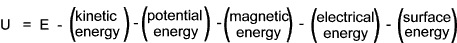Notes on the internal energy and the total stored energy and its relevance to the first law are provided on webpage Thermodynamic Laws

Energy is intangible ..It is not practical to measure the total stored energy of a body, substance or system.  Its presence can only be recognised by its effects on its surrounding or connected materials or mechanisms.  A rock located at ground level in a normal environment does not seem to possess any energy but it has sufficient potential energy to destroy a creature standing below it in a deep hole: it has sufficient kinetic energy in the form of heat to melt ice: it may have sufficient chemical energy to heat a house ( if it is coal): it may have sufficient nuclear energy to destroy a city.....

Heat

Heat is the energy form which is transferred by a difference in temperature.   If a body at 50 deg C is positioned in a fluid at 10 deg C.   The fluid temperature rises as heat is transferred from the body.   (At the microscopic level the energy possessed as a result of its temperature is kinetic energy of the molecules and atoms.)

System

In thermodynamics a system is a 3D region in space under study.   A system can be an isolated, adiabetic, closed system or an open system and it is surrounded by a defined boundary.. The outside of the boundary is called the surroundings

For isolated system matter and energy do not cross the boundary..it is not influenced in any way by the part of space which is external to the system boundaries.

For adiabetic systems matter and heat do not cross the boundary..(energy in the form of work can cross the boundary)

For a closed system a fixed amount of matter which is enclosed by a boundary.   Only heat and work can be transferred across the boundary.

For an open system matter, heat and work flow across the boundary.

A simple system is a system that does not contain any internal adiabatic, rigid and impermeable boundaries and is not acted upon by external forces.

A composite System is a system that is composed of two or more simple systems.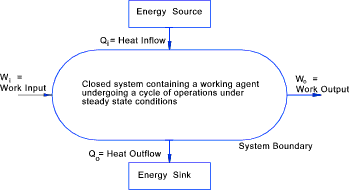Closed System

Boundary

The boundary encloses a system and may be real (physical) or imaginary.

A real boundary may be fixed e.g. a gas in a bottle, or movable e.g a gas in a cylinder which is compressed or expanded by movement of a piston.

An imaginary boundary is one not based on a physical control surface e.g. an engine could be analysed as a system contained within an imaginary boundary.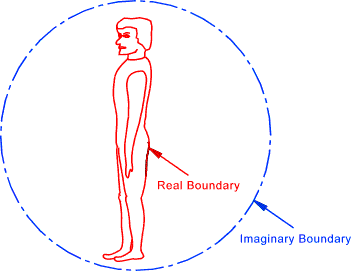Imaginary and Real Boundaries

Control Surface

An open system is often called a control volume and its boundary and most specifically the boundary under scrutiny is called the control surface.  Mass may flow across a control surface.  A cylinder piston in motion may be a control surface.

Surroundings

Everything outside a system boundary is called the surroundings.  Normally the term surroundings is restricted to those outside the system that in some way interact with the system or affect the behaviour of the system.

Thermodynamic State of a System

The condition of the system as characterized by the values of its state properties.

Stable equilibrium is a state in which the system is not capable of finite spontaneous change to another state without a finite change in the state of the surroundings.... Many types of equilibrium must be fulfilled -- thermal, mechanical, phase (material) and chemical.

State postulate: The equilibrium state of a simple closed system can be completely characterized by two independently variable properties and the masses of the species contained within the system.

Property ..A characteristic of a system

In the context of a thermodynamic system a "state property" identifies a condition of the system (or substance) at an equilibrium state which is independent of the path of the process by which the state has been reached.  The change in the pressure (P) or the temperature (T) of a system between two equilibrium states is the same for all paths and therefore the pressure and temperature are properties.  All properties of equilibrium states e.g. P,V,m and T are characteristics of the activities of large quantities of molecules..

Extensive properties are those properties with values representative of the sum of parts are called extensive properties e.g. m (mass), V (volume), (H) total enthalpy.

Intensive properties are those with values not representative of the sum of parts are called intensive properties e.g. the temperature (T). Pressure is also an intensive property

Specific properties are extensive properties per unit mass.   Specific properties are intensive.

Some Typical Thermodynamic (extensive) properties of fluids include..

 (V) Volume (m3) (m)Mass (kg) (H)Enthalpy (J) (U) Internal Energy (J) (S) Entropy (J)

Some Typical Thermodynamic (intensive) properties of fluids include..

 T temperature [K] ρ density [kg/m3] cp specific heat at constant pressure [J/kg�K] cv specific heat at constant volume [J/kg�K] μ dynamic viscosity [N/m2s] ν kinematic viscosity [m2/s] k thermal conductivity [W/m�K] α thermal diffusivity [m2/s] β volumetric thermal expansion coefficient [K-1] h specific enthalpy [J/kg] s specific entropy [J/kg�K] G gibbs free energy [J/kg]

A primitive property can in principle be specified by describing an simple operation or test to which the system is subjected.e.g using mechanical measurements ... pressure, volume, thermometric temperature (T) and heat capacity.

A derived property is a property that is mathematically defined in terms of primitive properties.

Process

A thermodynamic process may be defined as the progress of a thermodynamic system proceeding from an initial state to a final state.   The series of states the substance or system experiences as it progresses through the process is called the path of the process.

Typically, a thermodynamic process can be characterised, according to what system property e.g.temperature, pressure, or volume, etc., are held fixed.   Furthermore, it is useful to group these properities into pairs, in which the variable held constant is one member of the pair.   The six most common thermodynamic processes are shown below:

 An isobaric process occurs at constant pressure...ΔP= 0 An isochoric process, or isometric process, occurs at constant volume...ΔV= 0 An isothermal process occurs at a constant temperature...ΔT= 0 An isentropic process occurs at a constant entropy...ΔS= 0 ( Adiabetic and reversible) An isenthalpic process occurs at a constant enthalpy...Δh= 0 An adiabatic process occurs without loss or gain of heat...ΔQ= 0 A cyclic process occurs with same initial and final states A polytropic process has the relationship pV k = constant

There are a number of thermodynamic process types encountered by engineers including non-flow, steady flow, semi-flow and unsteady flow.   These are described as follows:

 Non flow processes are those involving no flow of matter across the system boundaries Steady flow processes involve fluid entering and leaving the system control volume these flows do not change with time and the internal energy of the control is also fixed in the time period under consideration A Semi-flow process involves fluid flow into a control volume which may be rigid charging a gas bottle or flexible -blowing up a balloon An unsteady flow process is one with a variable internal energy- i.e changing liquid level in a boiler

Reversible /Irreversible Process

If the substance or system passes through a continuous series of equilibrium states in progressing through the process as it receives or rejects energy it is referred to as a reversible process.  The path of this theoretical process is generally shown on diagrams as a full line.  If the process is reversed, in the thermodynamic sense, it would leave no trace of itself.

In the real world there are no reversible processes..All processes are irreversible and are shown on diagrams as broken lines...Factors which make a process irreversible include friction, unrestrained gas expansion, heat transfer across finite temperature difference, mixing,chemical reactions etc. etc...

Ideal Gas

An ideal gas is one that behaves according according to the assumptions that the volume of a gas molecules can be discounted and that molecules do not attract each other.   Various relationships have been arrived at for and ideal gas including Charles Law, Boyle's Law and Avogradros Law. ref. Ideal Gas .. An ideal gas conforms to the ideal gas law

Pv = nRT

 P = Pressure = (Pa) N/m 2 v = Volume = m 3 T = Absolute Pressure = deg Kelvin R = Universal Gas Constant = 8,314 J /mole.K = 8 314 J /kmole.K n = Number of moles

The laws and rules for ideal gases are only reasonably accurate for gases at low pressures and moderately high temperatures...At pressures around 1 bara or less the ideal gases are generally reasonably accurate for real gases.

Perfect Gas

A perfect gas is an ideal gas for which the values of the specific heats cp and cv are assumed constant.   ref. Properties This is an idealisation of the behaviour of real gases at low pressures e.g. oxygen and nitrogen at atmospheric pressure and ambient temperature.

Semi-Perfect Gas

Semi perfect gases are those subject to a wide variation of temperature such that it cannot be assumed that the specific heats are constan.   Semi Perfect gases are ideal gases for which the values of the specific heats cp and cv are allowed to vary as function of T alone.   ref. Properties.

Thermal Efficiency

The thermal efficiency is a measure of the success of a thermodynamic process.   It is generally expressed in simple terms as the ratio of the energy desired and the energy expended.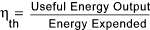Typical efficiencies include

 Automobile IC engines ...12-15% Gas Turbine ...12-16% Steam Power Plant ...38-41% Solar Cell ...15% Fuel Cell ...40-60% Electric Motors ...